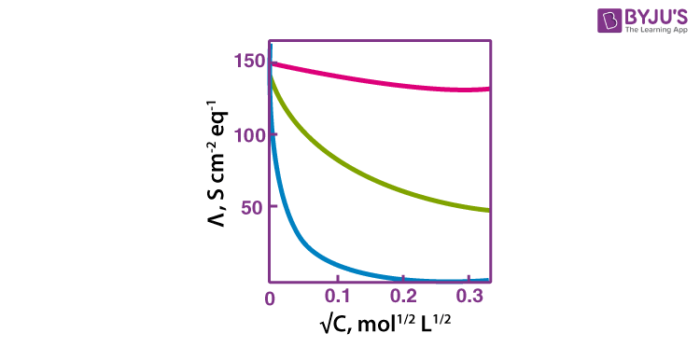# Variations Of Molar Conductivity With Concentration

Specific conductivity or conductivity of an electrolytic solution at any given concentration is the conductance of unit volume of solution kept between two platinum electrodes with a unit area of cross-section and at a distance of unit length. Conductivity decreases with decrease in concentration as the number of ions per unit volume that carry the current in a solution decrease on dilution.

## Molar conductivity

Molar conductivity of a solution at a given concentration is the conductance of volume V of a solution containing one mole of electrolyte kept between two electrodes with an area of cross-section A and distance of unit length.

Ʌm = К/c

Here,

c = concentration in moles per volume
К = specific conductivity
Ʌ= molar conductivity.

As the solution contains only one mole of electrolyte, the above equation can be modified as:

Ʌm =К V

Molar conductivity increases with decrease in concentration as the total volume, V, of a solution containing one mole of electrolyte also increases. Upon dilution, the concentration decreases. When the concentration approaches zero, the molar conductivity of the solution is known as limiting molar conductivity, Ë°m. Variation of molar conductivity with concentration is different for strong and weak electrolytes.## Variation of molar conductivity

• ### With concentration for strong electrolytes:

For strong electrolytes, the molar conductivity increases slowly with the dilution. The plot between the molar conductivity and c1/2 is a straight line having y-intercept equal to Ë°m. The value of limiting molar conductivity, Ë°m can be determined from the graph or with the help of Kohlrausch law. The general equation for the plot is given as:

For strong electrolytes, the molar conductivity increases slowly with the dilution. The plot between the molar conductivity and c1/2 is a straight line having y-intercept equal to Ë°m. The value of limiting molar conductivity, Ë°m can be determined from the graph or with the help of Kohlrausch law. The general equation for the plot is given as:

Ʌm = Ë°m -Ac1/2

Where -A is a constant equal to the slope of the line. For a given solvent, the value of “A” depends on the type of electrolyte at a particular temperature.

• ### With concentration for weak electrolyte:

For weak electrolytes, the graph plotted between molar conductivity and c1/2 (where c is the concentration) is not a straight line. Weak electrolytes have lower molar conductivities and lower degree of dissociation at higher concentrations which increases steeply at lower concentrations. Therefore, limiting molar conductivity, Ëm° cannot be obtained by extrapolation of molar conductivity to zero concentration. Hence, we use Kohlrausch law of independent migration of ions for determining to limit molar conductivity, Ëm° of weak electrolytes.

For a detailed discussion on the variation of molar conductivity with the concentration of electrolyte, download BYJU’S – The Learning App.

### Define molar conductivity.

The conducting power of all the ions which is produced by one mole of an electrolyte and dissolving it in the solution.

### How does molar conductivity depend on temperature?

As temperature increases, the molar conductivity also increases.

### Does molar conductivity vary with dilution? How?

Yes, molar conductivity varies with dilution. It increases with dilution.

### Difference between conductivity and specific conductivity.

Specific conductance also termed as conductivity, specific electrical conductance or electrical conductivity is an estimate of the ability of water to conduct electricity.